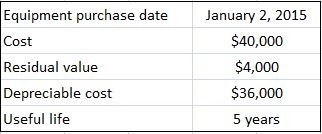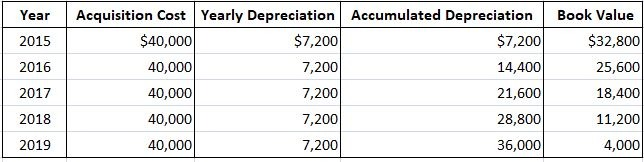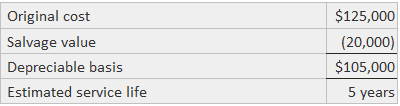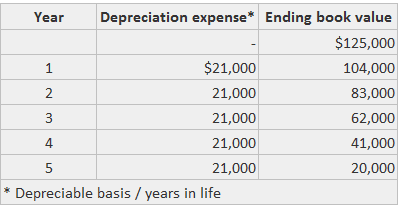## What Is the Straight-Line Method?

Straight-line depreciation is the simplest of the various depreciation methods. Under this method, yearly depreciation is calculated by dividing an asset’s depreciable cost by its estimated useful life.

By a large margin, the most easily understandable and widely-used depreciation method is the straight-line method. In this approach, an equal amount of depreciation is assigned to each year in the asset’s service life.

Under the straight-line method, the depreciable basis is divided by the number of years in the asset’s life in order to determine the average annual expense. The straight-line method is best applied when the cost assigned to each year is the same.

## Formula

Depreciation expense in the straight-line method is calculated as follows:

Depreciation = (Cost of the asset – Residual value) / Number of years of useful life

You can apply the straight-line method to calculate depreciation on assets that are used fairly uniformly over all the years of their useful life.

## Straight-Line Method of Depreciation: Explanation

To explain the straight-line method of depreciation, consider the following data:Using the above data, yearly straight-line depreciation is \$7,200, which is calculated as follows:

(Cost – Salvage value) / Useful life = (\$40,000 – \$4,000) / 5 = \$7,200

When the straight-line method is used, the depreciable cost of the asset is spread evenly over its life, in this case at a uniform rate of 20% (1 / 5 = 20%).

Therefore, depreciation expense is the same each year, and by the end of the fifth year, the asset’s book value has been reduced to its estimated residual value of \$4,000.

Even if the equipment is still used past the fifth year, it is left at its book value of \$4,000. These points are summarized in the following schedule:This example assumes that an entire year’s depreciation is taken in the year of acquisition.

However, in the real world, companies purchase assets at different times during the year, and a full year’s depreciation need not be taken on a partial year’s usage.

Furthermore, depreciation is often calculated monthly or quarterly for the preparation of interim statements.

To illustrate how to calculate partial year’s depreciation, assume that in the above example, the asset was purchased on 1 April rather than on 2 January.

In this case, only 9 months of depreciation expense, or \$5,400 (\$7,200 x 9/12), is recorded on 31 December.

Straight-line depreciation is widely used due to its simplicity and the fact that it allocates an equal amount of expense to each period of the asset’s life.

Even though from a conceptual perspective, straight-line depreciation is most appropriate for assets that give up their benefits on a fairly uniform basis, management can use it regardless of the pattern that assets give up their benefits.

## Example 1

This example calculates the depreciation expense for an asset (see table below) using the straight-line method.The total amount of depreciation is \$105,000 divided by five years (i.e., \$21,000 per year).## Example 2

Suppose an asset costs \$50,000 and has a useful life of 10 years. The depreciation expense for each year is:

50,000 / 10 = \$5,000

In this example, the depreciation rate can also be specified in terms of a percentage. A 10-year life indicates a depreciation rate of 1/10 or 10%.

Also, since the asset had an estimated useful life of 10 years, the depreciation expense each year was 1/10 of the depreciable amount.

In actual practice, when it becomes difficult to estimate the residual value or the calculation of residual value is not material, depreciation can be calculated by simply dividing the cost of the asset by the number of years of estimated useful life.

### What is the straight-line depreciation formula?

Depreciation = (Cost - Salvage Value) / Useful life

### What is the straight-line method of asset depreciation?

A straight-line basis is a method of calculating Depreciation and amortization. It is also known as straight line Depreciation. This formula for calculating asset value involves dividing the cost of an asset by its useful life, resulting in a constant rate of Depreciation per period.

### When should you use the straight-line method?

Straight-line Depreciation is a method of allocating the cost of a depreciating asset evenly over its useful life. It is most appropriate when an asset's value decreases steadily over time at around the same rate.

### Why is the straight-line method important?

The straight-line Depreciation method makes it easy for you to calculate the expense of any fixed asset in your business. It allows you to reduce the value of a tangible asset, thereby lowering your total expenses (and taxes).

### What is the difference between straight-line depreciation and declining balance depreciation?

Using the straight-line method, an asset's value is depreciated uniformly over its useful life, while a declining balance approach allocates more Depreciation in the early years than in the late years.

True is a Certified Educator in Personal Finance (CEPF®), a member of the Society for Advancing Business Editing and Writing, contributes to his financial education site, Finance Strategists, and has spoken to various financial communities such as the CFA Institute, as well as university students like his Alma mater, Biola University, where he received a bachelor of science in business and data analytics.

To learn more about True, visit his personal website, view his author profile on Amazon, his interview on CBS, or check out his speaker profile on the CFA Institute website.